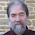Winner of the New Statesman SPERI Prize in Political Economy 2016

## Sunday, 13 April 2014

### Secular Stagnation and Three Period OLG

For macroeconomists. This post is a kind of introduction to the new paper on secular stagnation by Eggertsson and Mehrotra. As usual, any misinterpretations are my fault.

A basic idea behind secular stagnation is that the natural real rate of interest might become negative for a prolonged period of time. A simple way to model this would be to allow the steady state real interest rate to become negative. That cannot happen in basic representative agent models, where the steady state real interest rate (absent growth) is given by

1+r = 1/b

where b<1 is the utility discount factor. With population growth (at rate = n) this becomes

1+r = n +1/b

Note that a fall in n will reduce the real interest rate, which is a useful result if we want to relate secular stagnation to falling population growth, but rates cannot fall below the rate of time preference.

In a standard two period OLG model we have more flexibility. If agents only work in the first period, then they need to save in that period to be able to smooth consumption between their working lives and retirement. If we allow them to do that through investing in capital, and if α is the exponent on capital in a Cobb Douglas production function, then with log utility the real interest rate in steady state is given by

r = k + kn        where              k = α(1+b)/b(1- α)

If one period is about 25 years, then b could be 0.5 (annual b = 0.973), and with α = 0.4 then k=2. So now the impact of a fall on population growth on the real interest rate is magnified, but the steady state real interest rate is also likely to be above the representative agent case. (If n=0 and b = 0.5, then we have r=1 and r=2 respectively. For a 25 year period this would correspond to annual interest rates of around 2.8% and 4.5%.)

In a three period OLG setup, we can have saving without capital. The middle aged work (receiving income Y), and they lend to the young, and in retirement get paid back by the now middle aged. Suppose, however, that because of some credit friction the amount the young can borrow gross of interest payments is fixed at D, and let d=D/Y<1. The middle aged would like to lend them enough to smooth consumption, so the supply of loans in steady state is (given log utility)

b (Y-D)/ (1+b)

where Y-D is middle age income net of repaying loans taken out when young. The demand for loans is

D (1+n)/(1+r)

The borrowing limit is gross of interest, so with no population growth actual borrowing is D/(1+r). With population growth there are more of the young than middle aged, so we need to scale up loan demand accordingly. The real interest rate equates demand and supply, which implies

1+r = j + jn       where              j = (1+b)d/b(1-d)

Now if d is small, j could be less than one, which reduces the sensitivity of interest rates to population growth, although a fall in population growth still reduces rates. However this also means that the gross interest rate (1+r) could be less than one, so the steady state real interest rate could be negative.

The middle aged need to save for retirement, but the only way they can do this is by lending to the young. The higher the real interest rate, the less the young can borrow because of the credit friction. In that situation, the real interest rate could easily be negative, because only then will the young be able to borrow enough to allow the middle age to consumption smooth when they retire.

The key result that Eggertsson and Mehrotra explore is that a credit crunch - a fall in D - could lower real interest rates into negative territory, and could therefore generate secular stagnation. They consider how inequality could be incorporated into the model, and then embed the model in a nominal framework. Nominal wage rigidity is added (using a similar mechanism to that in the Schmitt-Grohe and Uribe paper I discussed here), and the implications for monetary and fiscal policy explored. So I have only touched on the paper here, but as this three period OLG set-up is not standard I thought this post might be useful.

1."The higher the real interest rate, the less the young can borrow because of the credit friction."

I'm glad you pointed this out, because in this model cutting interest rates raises consumption for no other reason than that, for an assumed cap on principal plus interest, lenders can then lend a higher principal (apart from a temporary transitional effect when rates change). As a role for the interest rate, this is rather unconventional and they perhaps should have said more about it. It's also worth noting that if you instead assume that the credit cap applies to principal net of interest, that the relationship between interest rates and income is reversed.

2.Nick Rowe
1.2.